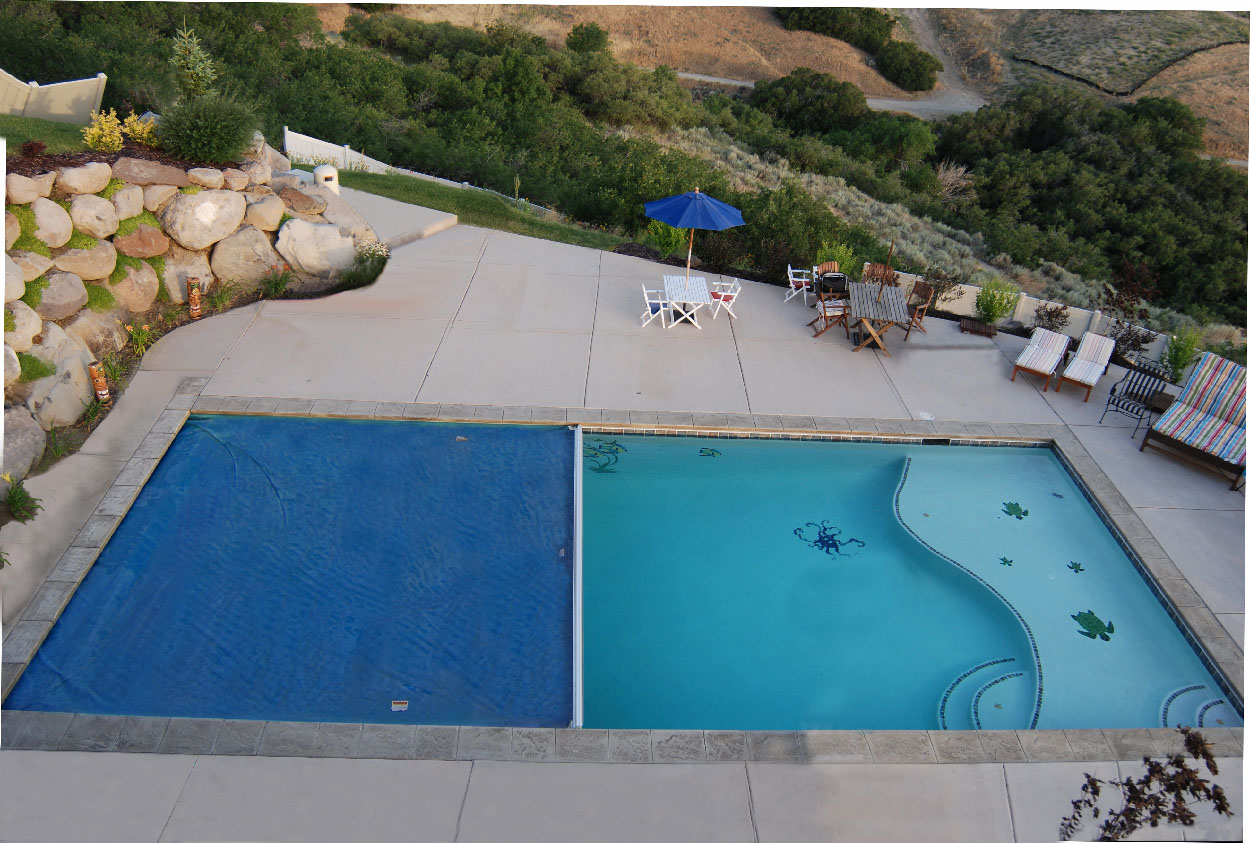# Introduction to the Concept of Perimeter

Aside from Algebra problems, the Civil Service Examination also contains geometric problems. In this post, I am going to introduce you to the concept of perimeter.

The perimeter of a polygon is the total distance around it. If you have a rectangular pool, for example, and you start walking from one corner along its edge (as near as you can), until you reach the place where you started, then the distance you have walked is its perimeter.Therefore, if you have a rectangular pool with length 20 meters and width 15 meters, then the perimeter is$20 + 20 + 15 + 15 = 70$ meters. Remember, there are two long sides and two short sides, so you have to add the numbers twice.  Therefore, if a rectangle has a given length and width, it’s perimeter is twice it’s length plus twice it’s width. Or, we can put the equation$P_t = 2l + 2w$

Where$P_t$ is the perimeter of the rectangle and$l$ and$w$ are its length and width respectively.

Since the idea of perimeter is to add all the distance around the polygon, this means that a square with side 5 has perimeter$5 + 5 + 5 + 5 = 4(5) = 20$.

This means that the perimeter of a square$P_s$ with side s$k$ is given by the formula$P_s = 4k$

We don’t really need to derive all the formula once you already know the concept since all you have to do is to add all the side lengths.

Perimeter of a Circle

The circle has also a perimeter, but we call it circumference. If you have a circular garden and you wall around it one time, then the distance you have walked is its circumference.The perimeter of a circle is$2 \pi r$ where$r$ is it’s radius (distance from the center to the circle) and$\pi$ is approximately equal to$3.14$.  If you want to ask where did the formula come from, it is beyond the scope of this blog to derive it (I have written a derivation about it in Filipino).

Now that you already know the concept of perimeter, we will solve some problems about it in the next post.